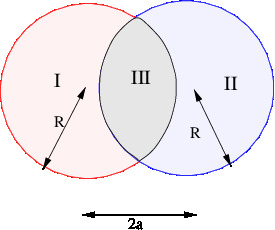# [YMP/EM-02010] Electric Field of Overlapping Uniformly Charged Spheres

For page specific messages
For page author info
 Problem Two spheres, each of radius $R$ and carrying charge densities $+\rho$ and $-\rho$ respectively, are placed so that they partially overlap. The separation between the centers of the spheres is $D$. Show that the field in the region of overlap is constant and find its value.Solution
Let the centres of the two spheres be at $\vec{r}_1$ be the position vector of a point in the overlap region drawn from the center of the first sphere and $\vec{r}_2$ be the position vector of the same point from the center of the second sphere.
Using the result for the field of a unformly charged sphere, we get

the electric field in the overlap region due to the first sphere =  $\displaystyle \frac{\rho \vec{r_1}}{3\varepsilon_0}$
the electric field due to the second sphere is   $\displaystyle - \frac{\rho \vec{r_2}}{3\varepsilon_0}$

Thus the total electric field, using superposition principle,
$$E= \frac{\rho(\vec{r}_1-\vec{r}_2)}{3\varepsilon_0} = \frac{\rho \vec{D}}{3\varepsilon_0}$$
where   $\vec{D}=\vec{r}_1-\vec{r_2}$,is the separation between the centers of the two spheres.Thus the electric field is constant.

n
0

X